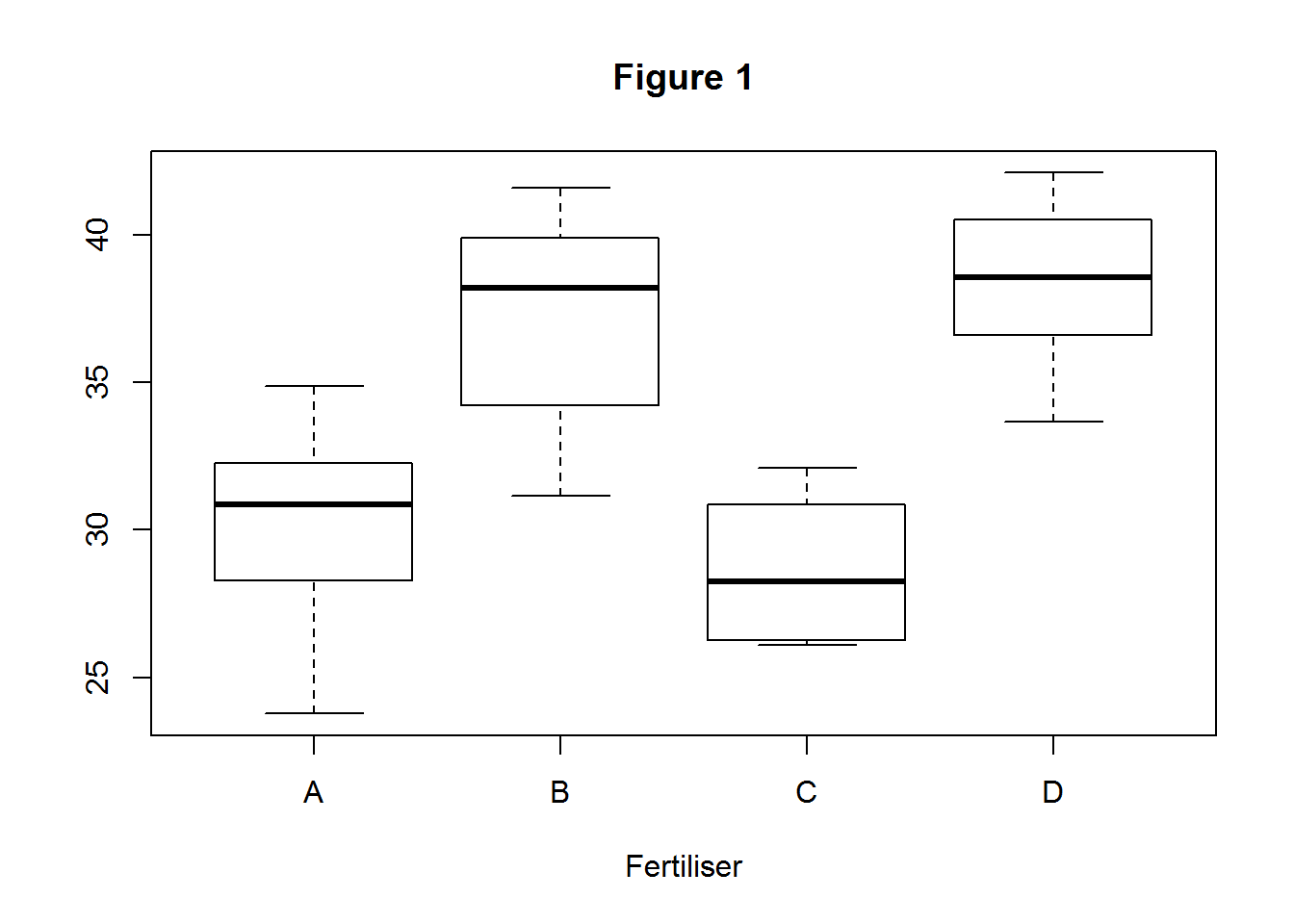### Principles of ANOVA

Suppose we are a turnip farmer who is interested in how different fertilisers affect the amount of vitamin C found in the leaves of our turnip plants.

We thus set up an experiment. Four different fertilisers are used on four different groups of leaves ($$N=8$$; i.e., 8 leaves in each group) and the vitamin C levels in the leaves are measured after some time period.

Read-in the “vitc_data.csv” data.

Figure 1 and Table 1 shows the results.

vitc <- read.csv("vitc_data.csv")
boxplot(vit~fert, main="Figure 1", xlab="Fertiliser", data=vitc)Fertiliser Vitamin C Mean, $$\bar{x_{i}}$$ Vitamin C Variance
1 30.18 (n = 8) 3.43
2 37.17 (n = 8) 3.75
3 28.62 (n = 8) 2.50
4 38.39 (n = 8) 2.78
Total $$\bar{x}=21$$ (n = 32) $$s^{2}=26$$

In order to test whether the means from each fertiliser group are different (or whether any differences are just due to sampling error) we use ANOVA. If there were only two groups, we could just use a t-test. But we cannot use multiple t-tests on multiple groups because this will inflate the chance of a Type I error.

ANOVA determines whether multiple treatments have significantly different means by dividing the mean square of the treatments ($$MST$$) by the mean square of the error ($$MSE$$, also denoted as $$\sigma_{e}^{2}$$).

The MST is obtained by dividing the treatment sum of squares ($$SST$$) by the degrees of freedom. The MST represents the variation between the treatment means.

The MSE is obtained by dividing the sum of squares of the residual error ($$SSE$$) by the degrees of freedom. The MSE represents the variation within each treatment.

Dividing the MST by the MSE gives F, which is a statistic that follows the F-distribution.

Under the null hypothesis, the F value should equal approximately 1; i.e., $$F=\frac{MST}{MSE}\approx{1}$$. This is because, under the null hypothesis, there are no treatment effects. However, there is always sampling error, and so $$MST\approx{MSE}$$ under the null hypothesis. In terms of our example, the leaves from each fertiliser group will possess the same Vitamin C concentration under the null hypothesis.

Under the alternative hypothesis, the F value should be greater than 1; i.e., $$F=\frac{MST}{MSE}>{1}$$. This is because at least one of the treatments has a significant effect on the outcome under the alternative hypothesis. But since sampling error is always present (i.e., the MSE is always incorporated into the MST), $$MST>MSE$$ under the alternative hypothesis. In terms of our example the leaves from at least one fertiliser group will possess a different Vitamin C concentration to one or more of the other fertiliser groups, under the alternative hypothesis.

### ANOVA by hand

So let’s first calculate the MST and MSE by hand. Firstly, we have to calculate the estimated overall mean, $$\hat{\mu}$$:

$\hat{\mu}=\frac{\mbox{sum of each vitamin C measurement}}{\mbox{number of measurements}}=\frac{\sum{y_{ij}}}{32}=33.59$

Now we have to calculate the estimated treatment effects $$\hat{A}_{i}$$, which are the differences between the estimated treatment means and the estimated overall mean:

$\hat{A}_{i}=Mean_{i}-\hat{\mu}$

That is,

$\hat{A}_{A}=30.18-33.59=-3.41$ $\hat{A}_{B}=37.17-33.59=3.57$ $\hat{A}_{C}=28.62-33.59=-4.97$ $\hat{A}_{D}=38.39-33.59=4.80$

Now we calculate the sums of squares. Firstly, the treatment sum of squares:

$\begin{eqnarray*} SST & = & \mbox{sum of squares between treatment groups}\\ & = & \sum\hat{A}_{i}^{2}\cdot\thinspace\mbox{number of measurements}\\ & = & \left(-3.41\right)^{2}\cdot8+\left(3.58\right)^{2}\cdot8+\left(-4.97\right)^{2}\cdot8+\left(4.80\right)^{2}\cdot8\\ & = & 577 \end{eqnarray*}$

Now the sum of squares within the treatment groups:

$\begin{eqnarray*} SSE & = & \mbox{sum of squares within treatment groups}\\ & = & \sum_{i}\sum_{j}\left(\mbox{individual value}_{ij}-\mbox{treatment mean}_{i}\right)^{2}\\ & = & \sum_{i}\sum_{j}\left(y_{ij}-\hat{y_{i}}\right)^{2}\\ & = & \sum_{j}\left(y_{Aj}-\hat{y_{A}}\right)^{2}+\sum_{j}\left(y_{Bj}-\hat{y_{B}}\right)^{2}+\sum_{j}\left(y_{Cj}-\hat{y_{C}}\right)^{2}+\sum_{j}\left(y_{Dj}-\hat{y_{D}}\right)^{2}\\ & = & 278 \end{eqnarray*}$

To calculate the degrees of freedom:

$\begin{eqnarray*} \mbox{We have 4 different treatments} & \rightarrow & df_{treat}=4-1=3\\ \mbox{We have 32 different measurements} & \rightarrow & df_{total}=32-1=31\\ df_{treat}+df_{error}=df_{total} & \rightarrow & df_{error}=31-3=28 \end{eqnarray*}$

And now the MST and MSE:

$\begin{eqnarray*} MST & \rightarrow & \frac{SST}{df_{treat}}=\frac{577}{3}=192.45\\ MSE & \rightarrow & \frac{SSE}{df_{error}}=\frac{274}{28}=9.94 \end{eqnarray*}$

The F-value is given by

$F=\frac{MST}{MSE}=\frac{192.45}{9.94}=19.37$

The critical F-value for degrees of freedom (3,28) and a probability level of 0.05 is $$2.95$$. Since $$19.37>2.95$$, then the null hypothesis has been rejected and it can be said that at least one of the treatments has a significant effect on the outcome. In other words, at least one of the fertilisers has an effect on the vitamin C concentration found in the turnip leaves.

### ANOVA using R

Read-in “vitc_data.csv” and create a new variable “vitc”

vitc <- read.csv("vitc_data.csv")

Use the aov function to calculate the analysis of variance of vitamin C concentration modelled by fertiliser type:

vitc_anova <- aov(vit ~ fert, data=vitc)
summary(vitc_anova)
##             Df Sum Sq Mean Sq F value   Pr(>F)
## fert         3  577.4  192.47   19.38 5.33e-07 ***
## Residuals   28  278.2    9.93
## ---
## Signif. codes:  0 '***' 0.001 '**' 0.01 '*' 0.05 '.' 0.1 ' ' 1

The $$MST=192.47$$, the $$MSE=9.93$$ and $$F=19.38$$, which are all very close to what was calculated by hand. In the ANOVA table returned by R we can see that $$p<0.05$$, and we conclude that at least one fertiliser treatment had an effect on the vitamin C concentration found in the turnip leaves.

However, ANOVA is only valid when certain assumptions are met. In the next lesson, we will learn how to test for those assumptions.